# Histogram

A visual interpretation of numerical data showing the number of data points falling within a specified range of values

## What is a Histogram?

A histogram is used to summarize discrete or continuous data. In other words, it provides a visual interpretation of numerical data by showing the number of data points that fall within a specified range of values (called “bins”). It is similar to a vertical bar graph. However, a histogram, unlike a vertical bar graph, shows no gaps between the bars.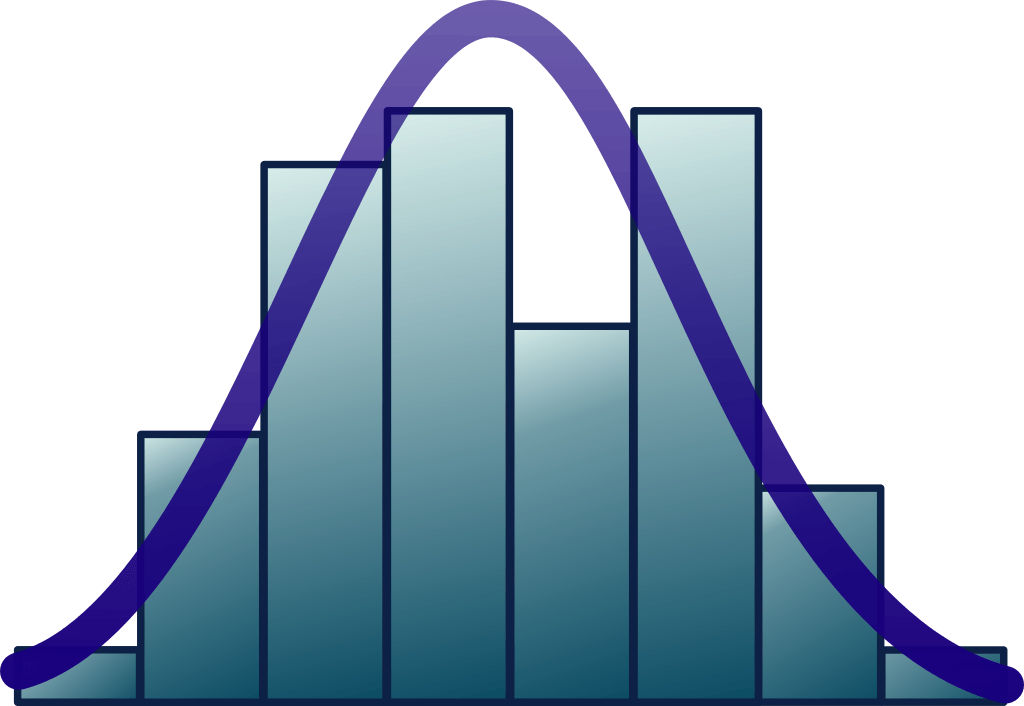### Parts of a Histogram

1. The title: The title describes the information included in the histogram.
2. X-axis: The X-axis are intervals that show the scale of values which the measurements fall under.
3. Y-axis: The Y-axis shows the number of times that the values occurred within the intervals set by the X-axis.
4. The bars: The height of the bar shows the number of times that the values occurred within the interval, while the width of the bar shows the interval that is covered. For a histogram with equal bins, the width should be the same across all bars.

### Importance of a Histogram

Creating a histogram provides a visual representation of data distribution. Histograms can display a large amount of data and the frequency of the data values. The median and distribution of the data can be determined by a histogram. In addition, it can show any outliers or gaps in the data.

### Distributions of a Histogram

A normal distribution: In a normal distribution, points on one side of the average are as likely to occur as on the other side of the average.A bimodal distribution: In a bimodal distribution, there are two peaks. In a bimodal distribution, the data should be separated and analyzed as separate normal distributions.A right-skewed distribution: A right-skewed distribution is also called a positively skewed distribution. In a right-skewed distribution, a large number of data values occur on the left side with a fewer number of data values on the right side. A right-skewed distribution usually occurs when the data has a range boundary on the left-hand side of the histogram. For example, a boundary of 0.A left-skewed distribution: A left-skewed distribution is also called a negatively skewed distribution. In a left-skewed distribution, a large number of data values occur on the right side with a fewer number of data values on the left side. A right-skewed distribution usually occurs when the data has a range boundary on the right-hand side of the histogram. For example, a boundary such as 100.A random distribution: A random distribution lacks an apparent pattern and has several peaks. In a random distribution histogram, it can be the case that different data properties were combined. Therefore, the data should be separated and analyzed separately.### Example of a Histogram

Jeff is the branch manager at a local bank. Recently, Jeff’s been receiving customer feedback saying that the wait times for a client to be served by a customer service representative are too long. Jeff decides to observe and write down the time spent by each customer on waiting. Here are his findings from observing and writing down the wait times spent by 20 customers: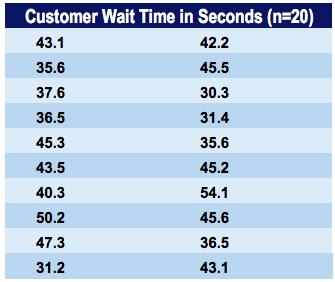The corresponding histogram with 5-second bins (5-second intervals) would look as follows: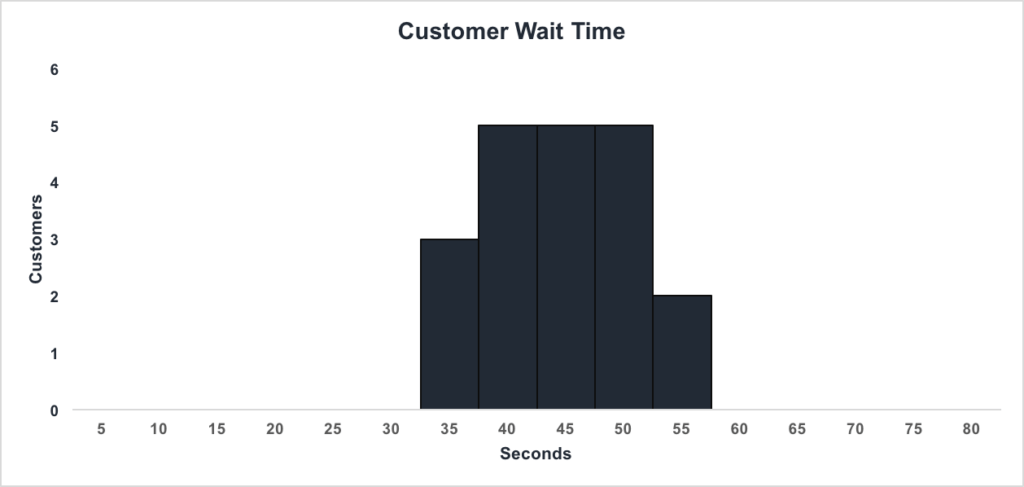We can see that:

• There are 3 customers waiting between 1 and 35 seconds
• There are 5 customers waiting between 1 and 40 seconds
• There are 5 customers waiting between 1 and 45 seconds
• There are 5 customers waiting between 1 and 50 seconds
• There are 2 customers waiting between 1 and 55 seconds

Jeff can conclude that the majority of customers wait between 35.1 and 50 seconds.

### How to Create a Histogram

Let us create our own histogram. Download the corresponding Excel template file for this example.

Step 1: Open the Data Analysis box. This can be found under the Data tab as Data Analysis:Step 2: Select Histogram: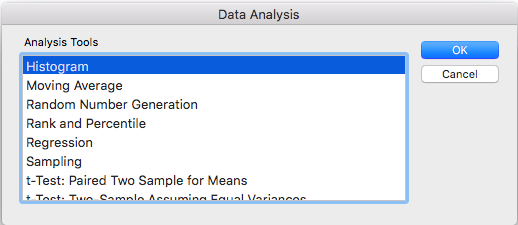Step 3: Enter the relevant input range and bin range. In this example, the ranges should be:

• Input Range: \$C\$10:\$D\$19
• Bin Range: \$F\$9:\$F\$24

Make sure that “Chart Output” is checked and click “OK”.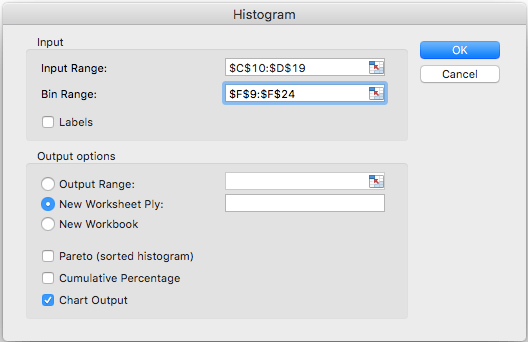CFI is the official provider of the global Financial Modeling & Valuation Analyst (FMVA)™ certification program, designed to help anyone become a world-class financial analyst. To keep advancing your career, the additional CFI resources below will be useful:

• Types of Graphs in Excel
• Dashboard Creation in Excel
• Excel Shortcuts PC & Mac
• List of Excel Functions

### Free Excel Tutorial

To master the art of Excel, check out CFI’s FREE Excel Crash Course, which teaches you how to become an Excel power user.  Learn the most important formulas, functions, and shortcuts to become confident in your financial analysis.

Launch CFI’s Free Excel Course now to take your career to the next level and move up the ladder!